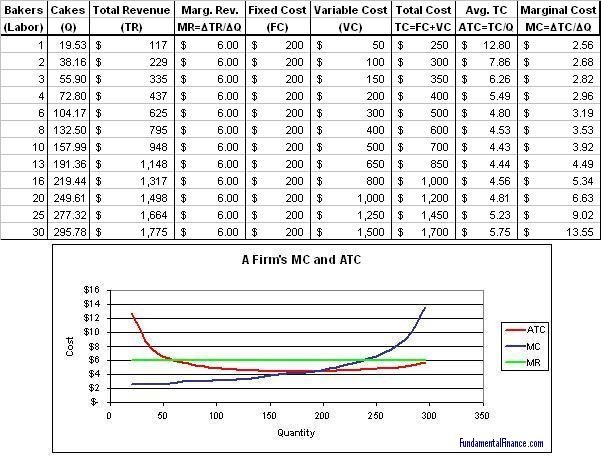## Marginal Cost and ATC

### Marginal Cost (MC) & Average Total Cost (ATC)

Total cost is variable cost and fixed cost combined.

##### TC=VC+FC
Now divide total cost by quantity of output to get average total cost.
##### ATC=TC/Q
Average total cost can be very handy for firms to compare efficiency at different output or when adjusting different factors of production.

Marginal cost is a concept that's a bit harder for people grasp. The "margin" is the end or the last. The marginal unit is the last unit. Think of marginal cost as the cost of the last unit, or what it costs to produce one more unit. It's hard to find exactly what the cost of the last unit is, but it's not hard to find the average cost of a group of a few more units. To find this, simply take the change in costs from a previous level divided by the change in quantity from the previous level.

##### MC = Change in TC / Change in Q
Take a look at the table below to see how marginal cost was computed. For example, the marginal cost when the quantity is 56 is \$2.82. This was computed by taking TC at 55.90Q (\$350) minus TC at 38.16Q (\$300) divided by 55.9Q minus 38.16Q (17.74Q).In other words, the marginal cost is factored into the average total cost at every unit. Because of fixed cost, marginal cost almost always begins below average total cost. As quantity increases, ATC will decrease and MC will increase. Eventually they intersect, then MC continues to increase and pulls ATC up after it.

A firm's marginal cost curve also acts as its supply curve. Read The Supply Curve article to get a more detailed explaination of why this is so.

Home
Accounting
Economics
Economics Articles
Economic Data
Economics Glossary
Macroeconomics
Economic Indicators
Fiscal Policy
Microeconomics
The Supply Curve
Price Elasticity
Fixed & Variable Cost
ATC & Marginal Cost
Marginal Revenue
Output Decision
Price Floor
Price Ceiling
Negative Externalities
Positive Externalities
Price Gouging
Sunk Costs
Game Theory
Game Theory Introduction
Nash Equilibrium
Extensive Form
Monopolies
Monopolies
The Sherman Act
The Clayton Act
Papers
Bootstrap Method
The CAPM Model
Euro Creation and Crisis
FX and Inflation in Pakistan
Poverty and Crime
General Finance
Mergers / Acquisitions
Money Market
Options
Real Estate
Retirement
Stocks
Other Personal Finance
Opinions / Essays
Excel